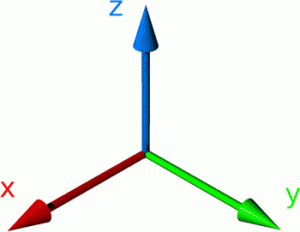# 357. It’s not square!

I do love a little challenge for A-level Further Maths students. They are often confident and very capable mathematicians, but occasionally overlook the small details. This challenge looks into which strategies students use when working with 3D vectors, lines and angles.

The most annoying thing? There is no single correct answer.

What is the investigation?

Students start with two points, create a line, construct two perpendicular lines and then join up the lines – did they create a square? How do you know? Justify it?

Download the instructions here: It’s not square (docx), It’s not square (PDF)

Skills required

• Distance between two points
• Equation of a line in three dimensions
• Scalar (dot) product

Solution/Discussion point

• Students need to use the same direction vector for both perpendicular lines too create a square
• The two new corners need to be n the same direction away from the original line (not one above and one below)
• It’s interesting to discuss what non-squares they made. Technology could be used to plot them in 3D.

# 211. Hidden Rectangle problem

Cool vectors can be exciting! They can describe the motion of a particle, they can represent the acceleration of a rocket, they can tell you about the angle an impact takes place at!Uncool vectors describe lines, they can intersect, they could be perpendicular, they could even describe skew lines in three-dimensions. Not quite as exciting. It isn’t difficult to see that revising standard C4 vectors can be a tad dull. How about an investigation? An investigation without an obvious answer. A question so simple that the answer is a single number. It’s the steps in between that make things interesting…

• I asked my A-Level class to find the area of a rectangle … simple so far, how is this worthy of C4?
• The rectangle is bounded by four vector equations … ok, points of intersection, line segment length, bit of Pythagoras there
• The vector equations are 3D … ooh, that makes it a bit harder
• There are eight equations to choose from … that’s mean, that means finding the angle between lines, checking for skewness, identifying parallel vectors
• There are plenty of ‘red herrings’ … now that is just unfair (great!)

The solution to the problem is a simple surd. If you do ‘Crack the Code’ or ‘Locked Box’ problems you could use the digits under the square root sign as your padlock code.

You can download the worksheet and teachers notes here: C4 Vectors Hidden rectangle (pdf)

Depending on the engagement/ability of the students this could take between 20 and 40 minutes. It would also make an easy to assess homework.